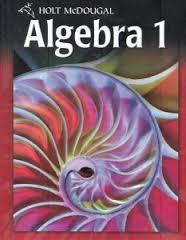2-1 HW Graphing And Writing Inequalities

7 Questions | Total Attempts: 217Settings• 1.
#30
• A.
• B.
• C.
• D.
• E.

Not here

• 2.
#32
• A.
• B.
• C.

Not here

• 3.
#36
• A.

All real numbers less than or equal to 23

• B.

All real numbers less than 23

• C.

All real numbers greater than 23

• D.

All real numbers greater than or equal to 23

• E.

No here

• 4.
#44 Define a variable; write an inequality. No graph of the solutions here.
• A.

H = height;

• B.

H = height;

• C.

R = # of rides;

• D.

Not here

• 5.
When graphing the solutions of an inequality, draw an open circle at the endpoint to...
• A.

Show that the number IS a part of the solution.

• B.

Show that the number IS NOT part of the solution.

• C.

Show that the inequality does not have a solution

• D.

Show that the solutions are "greater than or equal to" OR "less than or equal to".

• 6.
#26
• A.
• B.
• C.

Not hereBack to top